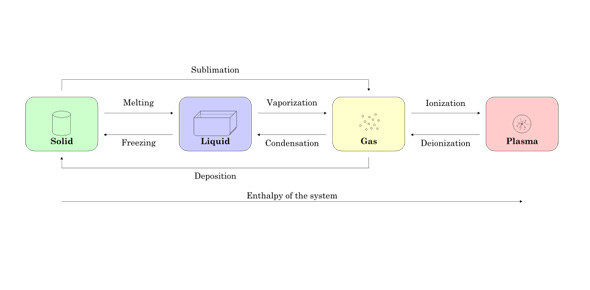# Test Your Knowledge On States Of Matter

12 Questions | Total Attempts: 103SettingsTake this scientific quiz and your knowledge on states of matter. Let's start this quiz now!

Related Topics
• 1.
What is a substance that is free to flow and has no particular shape, but does have a particular volume?
• A.

Solid

• B.

Liquid

• C.

Gas

• D.

Amorphous Solid

• 2.
What is a state of matter with particles that don't have a specific volume or shape?
• A.

Solid

• B.

Liquid

• C.

Gas

• D.

Amorphous Solid

• 3.
What is a substance that has a specific shape and size?
• A.

Solid

• B.

Liquid

• C.

Gas

• D.

Amorphous Solid

• 4.
In which state of matter are atoms moving around the most?
• A.

Solid

• B.

Liquid

• C.

Gas

• D.

Amorphous Solid

• 5.
What do you call a substance that has properties of both a solid and a liquid?
• A.

Newtonian Solid

• B.

Solid-Liquid

• C.

Solquid

• D.

Amorphous Solid

• 6.
What would I do to a substance to change it from a solid to a liquid?
• A.

Make it colder.

• B.

• C.

• D.

You can't change substances from solids to liquids.

• 7.
Which substance has the greatest viscosity?
• A.

Water

• B.

Oil

• C.

Vinegar

• D.

Corn Syrup

• 8.
What causes surface tension on a liquid?
• A.

The molecules on the surface stick together.

• B.

The liquid has a high density.

• C.

The molecules on the surface repel each other.

• D.

The cause of surface tension is a mystery.

• 9.
At what point does a gas become a liquid?
• A.

Boiling Point

• B.

Condensation Point

• C.

Freezing Point

• D.

Melting Point

• 10.
At what point does a solid become a liquid?
• A.

Boiling Point

• B.

Condensation Point

• C.

Freezing Point?

• D.

Melting Point

• 11.
At what point does a liquid become a gas?
• A.

Boiling Point

• B.

Condensation Point

• C.

Freezing Point

• D.

Melting Point

• 12.
At what point does a liquid become a solid?
• A.

Boiling Point

• B.

Condensation Point

• C.

Freezing Point

• D.

Melting Point